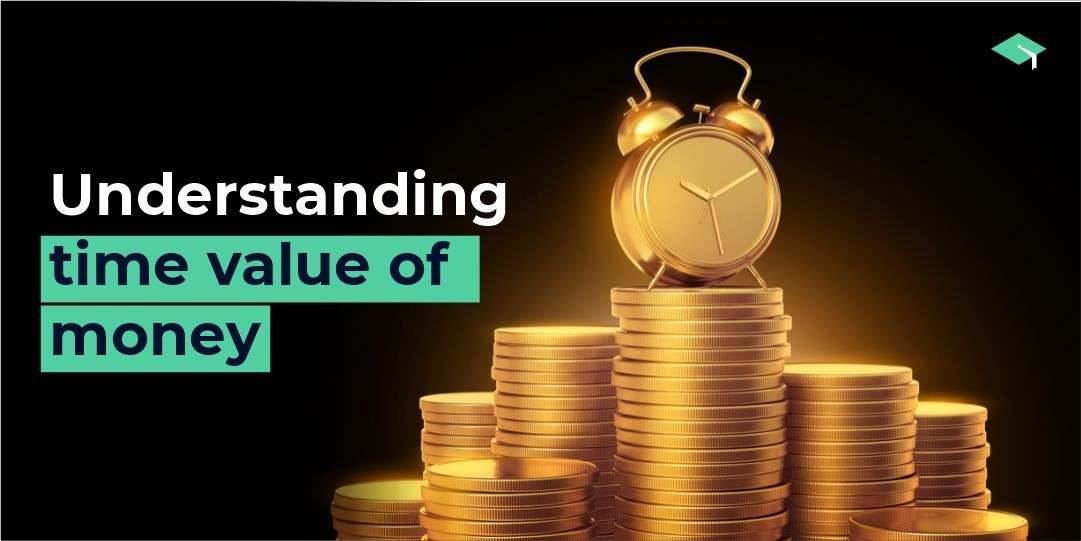# What is time value for money?

In the previous article, the focus was on why do you need to look beyond traditional investment options for your child’s education?. In this article, we will discuss the time value of money.

The idea of the time value of money tells us that money now is more valuable than money in the future because the money received now can be used for further investment, to obtain more returns.

Assume that you have lent Rs 2000 to your friend. Would you want him to pay you back today or tomorrow? The sensible answer is today because you’ll be able to put that money to work, to earn your potential gains.

Furthermore, with future money, there is a danger that the money might never be received, for whatever reason possible. The net present value or NPV is another term used for money’s time value.

The present value of future cash flow is calculated by dividing the future cash flow by a discount factor which incorporates the amount of time that will pass and the expected interest rate in the future.

## The time value for money and purchasing power

Inflation and buying power are two related concepts of the time value of money. Both these factors need to be considered, along with whatever rate of return might be obtained by investing the money.

While investing money, you must account for inflation and buying power because you need to subtract the inflation rate from the percentage gain you grow your investments with.

When you subtract the in rate of inflation return percentage, you get the real rate of return. If the inflation rate is higher than your investment return, you are losing money in terms of purchasing power even though your investment shows a nominal positive return.

For example, if you earn 10% of your investments and the rate of inflation is 12%, then, in reality, you are losing money.

#### The time value of money formula

FV = PV × (1+i)^n

Where FV = Future value of money,

PV = Present value of money, n = number of periods, and I = interest rate per period.

So, if we have to calculate the PV, the formula becomes PV=FV/ (1+i) ^n

And the calculation of FV requires the following formula FV= PV (1+i) ^n.

### Why does the time value of money matter?

Decision-makers can use the time value of money to assist them in choosing optimal alternatives because absolute rupee quantities across different periods have different values.

The time value of money equalizes options depending on timing. While comparing projects and businesses with different cash flows. The time value of money formula is frequently used.

Businesses can also utilize the time value of money to see if a project with an initial cash outlay and a later cash flow will be lucrative. Companies employ the time value for money for external reporting obligations.

Also, individual investors can better comprehend the actual value of their investments and liabilities over time by using the time value of money; the time value of money also helps to determine how much an investor will have in retirement.

It is important to note that the time value of money often ignores detrimental impacts to finance, such as negative interest rates or capital losses.

Negative growth rates that are unavoidable can be used in situations where losses are known.

### Some important points

1. The philosophy of the time value of money holds because money today can be invested and potentially grow into a more significant amount in the future.
1. A future cash flow’s present value is estimated by dividing it by a discount factor that considers the period that will pass and the predicted interest rates.
1. Multiplying the quantity of cash by a function of the expected rate of return over the predicted period yields the future value of a sum of money today.
1. The time value of money is used to make long-term financial decisions like whether to invest in a project or with which cash flow sequence is more advantageous than the other.

The applications of the concept are far-fetched in today’s financial world. Knowing it will be an excellent addition to your kitty of financial knowledge.